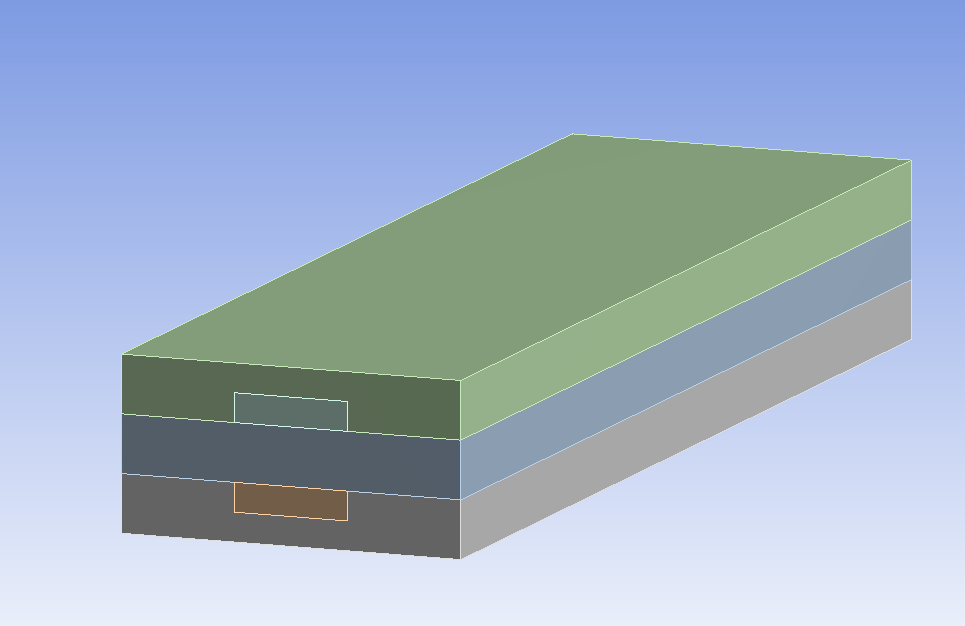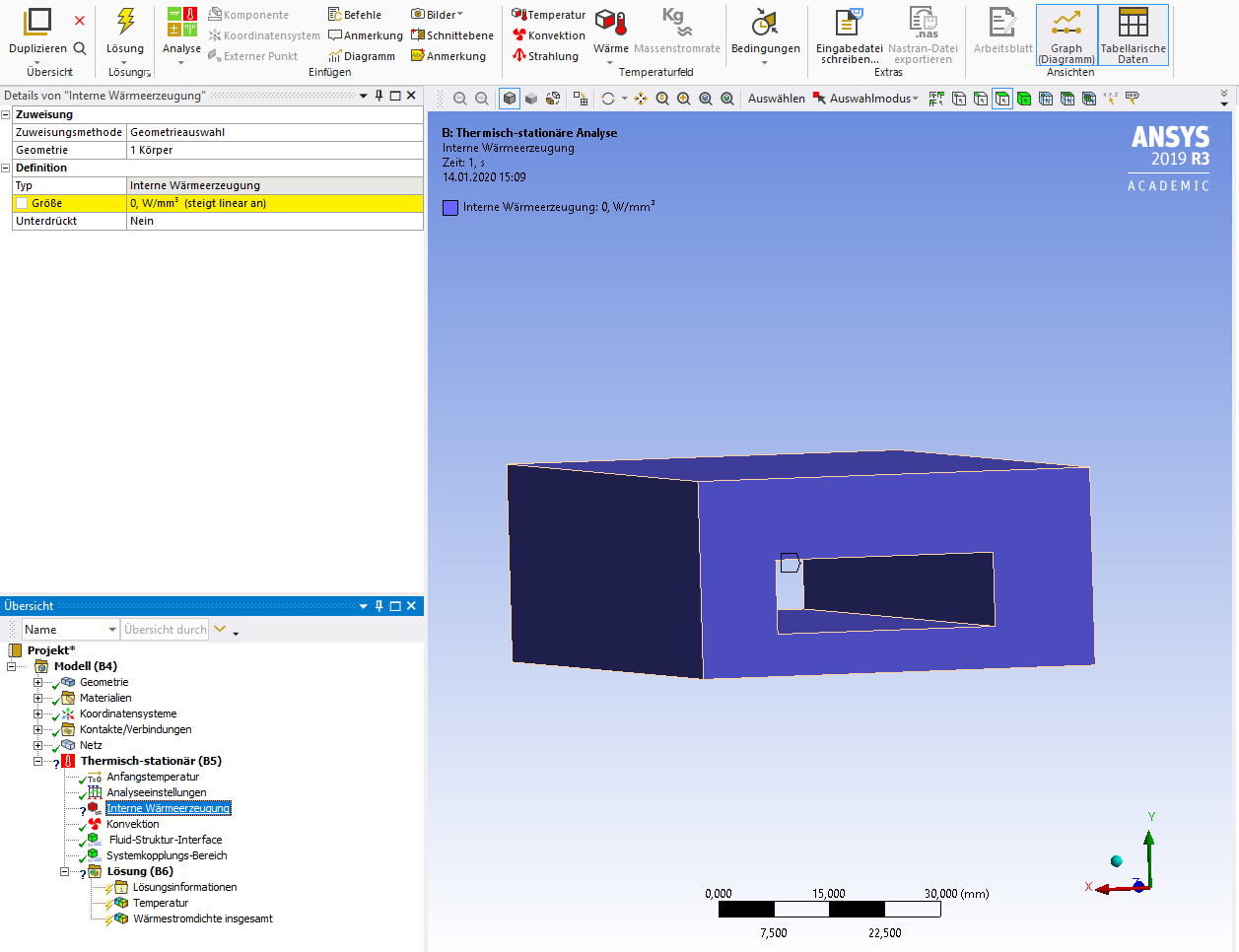# How to use a calculated heat source fluent udf as volumetric heat source transient thermal analysis?

• 87 Views
• Last Post 15 January 2020
Michael104 posted this 08 January 2020

Hi everyone,

i calculated the energy losses (chemical reaction/ohmic heating) of a fuel cell using a udf in fluent. Now i want to implement these energy losses as a volumetric heat source in the middle grey layer of the FC and calculate the generated temperature in the solid regions. Below is a little example how the geometry can look like. To calculate the Temperature i want to connect the fluent model with a thermal transient model to calculate the temperature.

Is it possible to model the volumetric heat source of the thermal transient model with a calculated heat using udf in fluent??

I know that that it is possible to implement a function as a volumetric heat source, but i don´t know how to use a function for heat source which is calculated in a udf in fluent.

Michael104 posted this 08 January 2020

Here is a litle image how the geometry can look like. And the middle layer is of course not grey, it is blue.

Thanksrwoolhou posted this 09 January 2020

You can use source term UDFs (and possibly expressions) to make the energy source a function of just about anything.

Michael104 posted this 14 January 2020

i think i understood what you mean. Yes i have modeled a udf energy source term as a function of temperature and pressure. Now i want to calculate the heat in the solid part, so i want to use the calculated heat source from fluent in a transient thermal model. I uses system coupling, but i don´t know how can i select the calculated energy source for the volumetric heat source in the termal model.

I can only set the volumetric heat source as constant, table, function... but how can i select the calculated udf from fluent.

Thanks for your helpMichael104 posted this 14 January 2020

above i wrote volumetric heat source, i mean of course internal heat source

rwoolhou posted this 14 January 2020

If the result from Mech doesn't alter the flow I'd look at transferring temperature to Mech rather than using system coupling. System coupling is designed for when the two interact.

Michael104 posted this 14 January 2020

Yes they interact, if i understand the workflow of ansys correct.

Maybe i can get some more details: Dependent on the temperature and the pressure of my solid part i want to calculate a internal heat source. The heat source heat my solid material, which gets cooled from a fluid flow, calculated in fluent. So i have a transient thermal model for the heat source and temperature distribution in solid and a fluid flow in fluent for a cooling channel. If i understand your answer correct, they should interact.

I don´t know how to set the internal heat source as a function of temperature and pressure?

Michael104 posted this 14 January 2020

Idea:

Is there a possibility to export in every iteration the amount of my calculated heat source f(T,p) in fluent to a chart and import this values in the thermal model as a internal heat source? In this case i would have a internal heat source as a function of temperature and pressure and a i think a one way FSI.

rwoolhou posted this 15 January 2020

Is the solid deforming under load/temperature/pressure?  If it's not then I'd solve it all in Fluent.Close